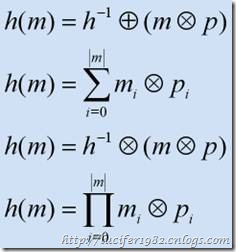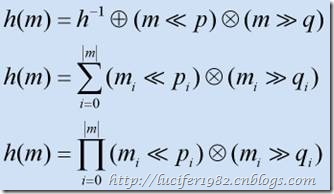# 数据结构 : Hash Table [I]

### 引子

Hash table，国内相当一部分书籍将其直译为哈希表，但博主本人喜欢称其为散列表。

10 namespace Lucifer.CSharp.Sample

11 {

12     class Program

13     {

14         public static void Main()

15         {

16             Hashtable table = new Hashtable();

17

18             //插入操作

19             table = "A";

21             table = "C";

22

23             //检索操作

24             string a = (string)table;

25             string b = (string)table;

26             string c = (string)table;

27

28             //删除操作

29             table.Remove(1);

30             table.Remove(2);

31             table.Remove(3);

32         }

33     }

34 }

10 namespace Lucifer.CSharp.Sample

11 {

12     class Program

13     {

14         public static void Main()

15         {

16             Dictionary<int, string> table =

17                 new Dictionary<int, string>();

18

19             //插入操作

20             table = "A";

22             table = "C";

23

24             //检索操作

25             string a = table;

26             string b = table;

27             string c;

28             table.TryGetValue(3, out c);

29

30             //删除操作

31             table.Remove(1);

32             table.Remove(2);

33             table.Remove(3);

34         }

35     }

36 }

### 散列函数

1. 数据分布。这是衡量散列函数生成散列值好坏的尺度。分析这个需要知道在数据集内发生碰撞冲突的数量，即非唯一的散列值。
2. 散列函数的效率。这是衡量散列函数生成散列值快慢的尺度。理论上，散列函数非常快。但是也应当注意到，散列函数并不总是保持 O(1) 的时间复杂度。

1. 加法和乘法。这个方法的主要思想是通过遍历数据，然后以某种计算形式来构造散列值。通常情况下是乘以某个素数的乘法形式。如下图所示：目前来说，还没有数学方法能够证明素数和散列函数之间的关系。不过在实践中利用一些素数可以得到很好的结果。
2. 位移。顾名思义，散列值是通过位移处理获得的。每一次的处理结果都累加，最后返回该值。如下图所示：8 public int JavaHash(string str)

9 {

10     int hashCode = 0;

11     for (int i = 0; i < str.Length; i++)

12     {

13         hashCode = 31 * hashCode + str[i];

14     }

15     return hashCode;

16 }

18 public int DJBHash(string str)

19 {

20     int hashCode = 5381;

21     for (int i = 0; i < str.Length; i++)

22     {

23         hashCode = ((hashCode << 5) + hashCode)

24             + str[i];

25     }

26     return hashCode;

27 }

29 public unsafe int DotNetHash(string str)

30 {

31     fixed(char* charPtr = new String(str.ToCharArray()))

32     {

33         int hashCode = (5381 << 16) + 5381;

34         int numeric = hashCode;

35         int* intPtr = (int*)charPtr;

36

37         for (int i = str.Length; i > 0; i -= 4)

38         {

39             hashCode = ((hashCode << 5) + hashCode +

40                         (hashCode >> 27)) ^ intPtr;

41             if (i <= 2)

42             {

43                 break;

44             }

45             numeric = ((numeric << 5) + numeric +

46                         (numeric >> 27)) ^ intPtr;

47             intPtr += 2;

48         }

49         return hashCode + numeric * 1566083941;

50     }

51 }

53 public int DEKHash(string str)

54 {

55     int hashCode = str.Length;

56     for (int i = 0; i < str.Length; i++)

57     {

58         hashCode = ((hashCode << 5) ^ (hashCode >> 27))

59                     ^ str[i];

60     }

61     return hashCode;

62 }

64 public int ELFHash(string str)

65 {

66     int hashCode = 0;

67     int numeric = 0;

68     for (int i = 0; i < str.Length; i++)

69     {

70         hashCode = (hashCode << 4) + str[i];

71         if ((numeric = hashCode & 0xF0000000L) != 0)

72         {

73             hashCode ^= (hashCode >> 24);

74         }

75         hashCode &= ~numeric;

76     }

77     return hashCode;

78 }

posted @ 2008-06-18 00:46 Angel Lucifer 阅读(...) 评论(...) 编辑 收藏